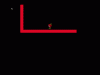# GMLTrouble with collision

#### Tiamat

##### Member
I was programming to make the character only move horizontally while in the air(thankfully got that to work), but the collision with the character and wall is extremely weird. Horizontally, the character floats from the platform a few pixels away, and as it approaches the wall vertically, the character just goes through. I'm lost as to what the reason might be. I'll post a video to show what I'm referring to and the code.

GML:
``````/// @description Player logic
mov_jump = keyboard_check(vk_space) || keyboard_check(ord("W")); //check for space bar, or W

var move = 0;

vsp += grv;

//jumping
if(mov_jump && jumpBuffer == 10)
{
vsp = -5.7;
jumpBuffer = 0;
inTheAir = true;
}

//can only move horizontially while in the air
if(inTheAir == true)
{
mov_right = keyboard_check(vk_right) || keyboard_check(ord("D")); //check for right arrow, or D
mov_left = keyboard_check(vk_left) || keyboard_check(ord("A")); //check for left arrow, or A

var move = mov_right - mov_left; //pos = right || neg = left
hsp = move * airMoveSpeed; //set horizontal speed in relation to 1 or -1
}
else
{
hsp = 0;
}

//horizontal collision
if(place_meeting(x + hsp, y, Wall_O))
{
while(!place_meeting(x + sign(hsp), y, Wall_O))
{
x += sign(hsp);
}
hsp = 0;
}
x += hsp;

//vertical collision
if(place_meeting(x, y + vsp, Wall_O))
{
while(!place_meeting(x, y + sign(vsp), Wall_O))
{
y += sign(vsp);
}
vsp = 0;
inTheAir = false;
}
y += vsp;

//animation
if(!place_meeting(x, y + 1, Wall_O))
{
sprite_index = Jumpe_jump_S;
image_speed = 0;

if(sign(vsp) < 0)
{
image_index = 0;
}
else
{
image_index = 1;
}

if(hsp != 0)
{
image_xscale = -(sign(hsp));
}
}
else
{
jumpBuffer = 10; //buffer refills

if(vsp == 0)
{
image_speed = 1; //set image speed to one for sprite animation
sprite_index = Jumpe_O;
}
}

if(hsp != 0)
{
image_xscale = -(sign(hsp)); //reverse image
}``````#### Slyddar

##### Member
Have you checked if the sprite collision mask is set correctly? Because changing direction causes the collisions to fail may indicate an incorrect mask, that also does not have the origin at the center.

I'd suggest you set the "collision mask" in the object to a single mask only, such as the idle sprite, and check the idle sprite has a correct mask. Also changing the image_xscale is not ideal if you have an even width mask, so a better way is to have a "facing" variable you change instead, and add a draw event with draw_sprite_ext() using facing as the image_xscale argument. This stops the mask being flipped, which can cause collision issues, and just draws the correct orientation instead.